# Important Centre of Mass Formulas for JEE Main and Advanced

Before we look at the centre of mass formulas lets us quickly look at what the concept means. The centre of mass of a body is defined as a point at which whole of the mass of the body appears to be concentrated. The centre of mass of few useful configurations is given below.

## Centre of Mass Formulas

1. A system of two point masses m1r1 = m2r2. The centre of mass lies closer to the heavier mass.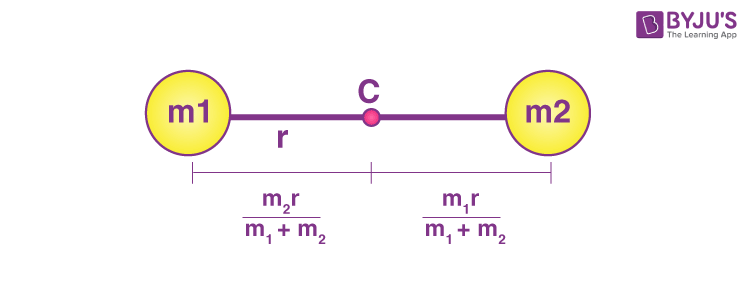2. Triangle (at the centroid)

yc=h/3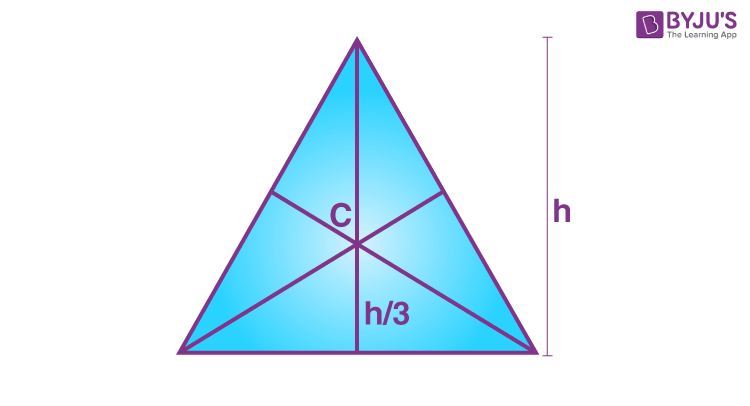3. A semi-circular disc

yc= 4r/3π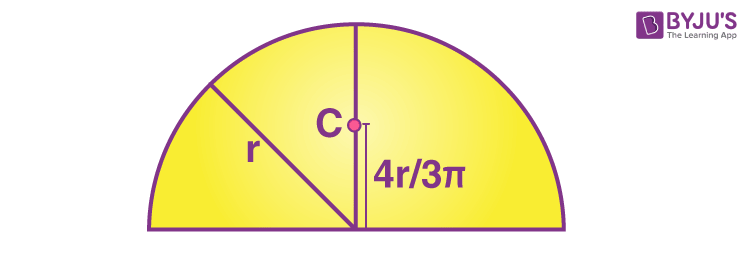4. Semicircular ring

yc= 2r/π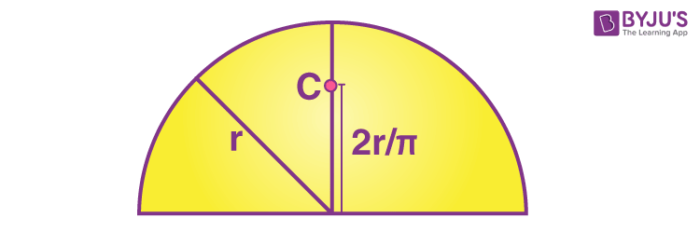5. Hemispherical shell

yc= r/2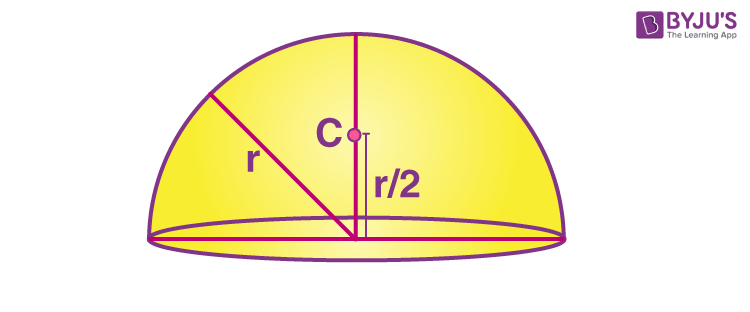6. Solid Hemisphere

yc =3r/8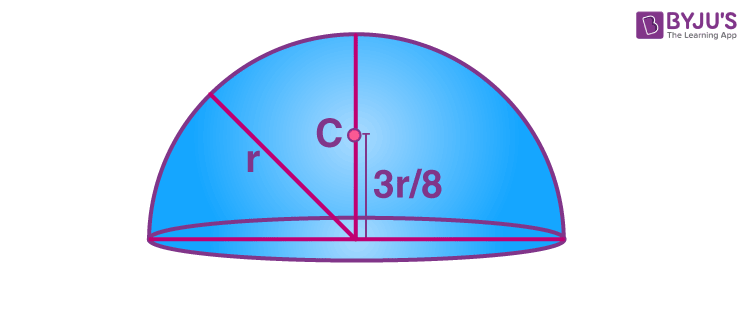7. Cone.

The height of the centre of mass from the base is h/4 for the solid cone and h/3 for the hollow cone.

## Centre of Mass Videos

### Problem illustration on Centre of Mass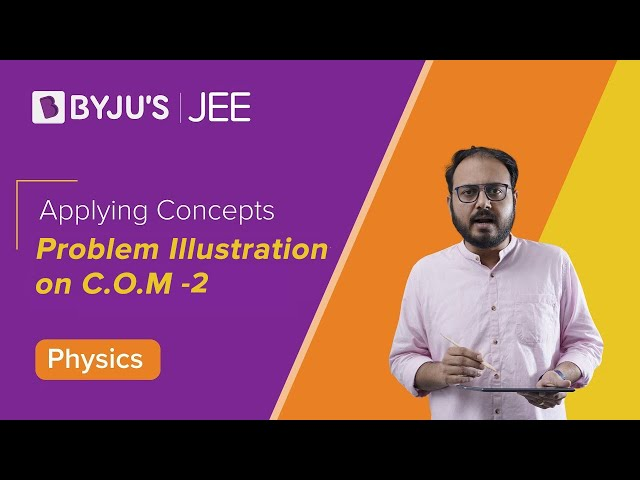## Motion of the Centre of Mass and Conservation of Momentum

### 1. Velocity of centre of mass

$\vec{V}_{cm}=\frac{m_{1}\vec{v_{1}}+m_{2}\vec{v_{2}}——-m_{n}\vec{v_{n}}}{M}$ $\vec{p}_{cm}=M\vec{v_{cm}}$

### 2. Acceleration of centre of mass

$\vec{a}_{cm}=\frac{m_{1}\vec{a_{1}}+m_{2}\vec{a_{2}}——-m_{n}\vec{a_{n}}}{M}$ $\vec{a}_{cm}=\vec{F}_{ext}{M}$

## Impulse

$\vec{J}=\int \vec{F}{dt}=\Delta\vec{p}$

## Collision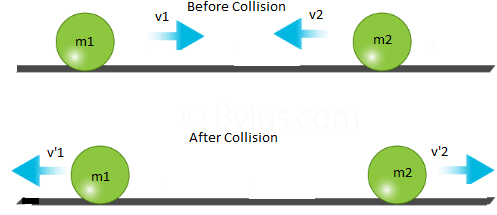Momentum conservation m1v1+m2v2 =m1v’1 + m2v’2

Elastic Collision= ½ m1v12 + ½ m2v22 = ½ m1v’1 2+½m2v’22

Coefficient of restitution

$e=\frac{-(v’_{1}-v’_{2})}{v_{1}-v_{2}}$

e=0, completely elestic collision

e=1, completely inelastic collision

If v2=0 and m1≪ m2 then v’1 = -v1

If v2=0 and m1 ≫ m2 then v’2 = 2v1

Elastic Collision with m1=m2 then v’1 = v2 and v’2 = v1

Test Your Knowledge On Important Centre Of Mass Formulas For Jee!